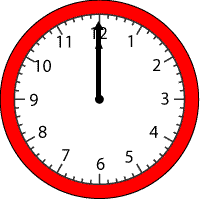#### You may also like### Times

Which times on a digital clock have a line of symmetry? Which look the same upside-down? You might like to try this investigation and find out!### Clock Hands

This investigation explores using different shapes as the hands of the clock. What things occur as the the hands move.### Ten Green Bottles

Do you know the rhyme about ten green bottles hanging on a wall? If the first bottle fell at ten past five and the others fell down at 5 minute intervals, what time would the last bottle fall down?

# Sweeping Hands

## Sweeping HandsIn $10$ minutes, through how many degrees does the minute hand of the clock sweep?

In $3$ hours, how many degrees does the hour hand of the clock sweep through?
If the minute hand goes through $180^{\circ}$, how many degrees does the hour hand sweep?

### Why do this problem?

This problem is a great context in which to apply knowledge of angles based on a full turn and it helps to consolidate analogue clock features too.

Having a large geared demonstration clock available would be a good idea to discuss this problem with the group.

### Key questions

Which is the minute hand and which is the hour hand?
How long does it take the hour hand do go a full circle ($360$ $^\circ$) round the clock?
How long does it take the minute hand do go a full circle round the clock?
How many degrees does the minute hand go through in one hour?
So many degrees does it go through in $15$ minutes? In $10$ minutes?
So, how many degrees does it go through in $5$ minutes?

### Possible extension

Learners could go on to either of these two problems - Watch the Clock or Two Clocks .

### Possible support

Suggest doing this problem, Two Clocks or possibly just exploring the interactivity to see how clocks move.